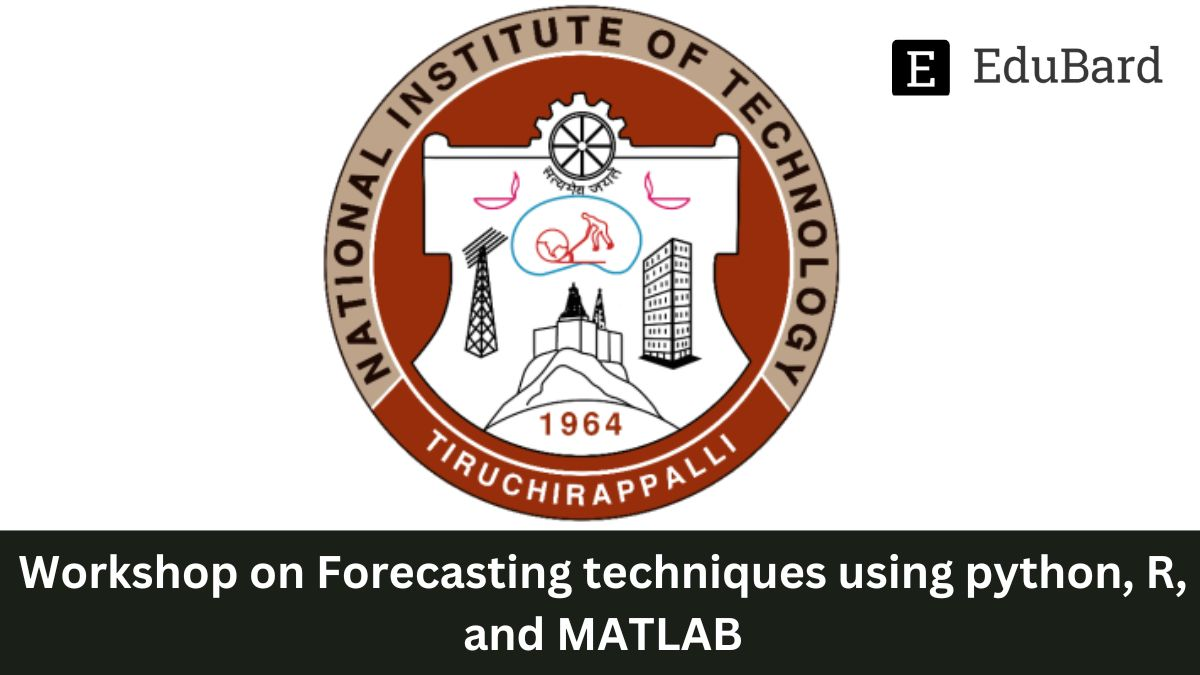# NIT Trichy | Workshop on Forecasting techniques using Python, R, and MATLAB, Apply Now!## Overview:

NIT Trichy is inviting applications for workshops on Forecasting techniques using Python, R, and MATLAB. Interested ones can apply.

This workshop introduces the participants to different traditional and advanced forecasting techniques using R, MATLAB, and Python. The workshop will have more practice-oriented sessions that will provide a working knowledge of Python, R, and MATLAB. After the workshop, the participants can apply the techniques with R, Python, and MATLAB to fulfill their research/academic needs.

## Target Audience:

• Students (UG& PG),
• Ph.D. Scholars
• Faculty &
• Working professionals

## Contents:

• Introduction to forecasting
• Installation- R, Python, and MATLAB
• Simple and multiple regression
• Simple and multiple regression lab
• ARDL Theory
• ARDL-LAB
• Vector Autoregression (VAR)
• VAR-LAB
• Exponential Smoothing Methods & Simultaneous-Equation
• Exponential Smoothing Methods & Simultaneous-Equation LAB
• AR, MA, and ARIMA
• AR, MA, and ARIMA- LAB
• ARCH & GARCH I
• ARCH & GARCH II
• ARCH & GARCH-LAB I
• ARCH & GARCH-LAB II
• Introduction to ANN
• Forecasting using ANN- LAB
• Hands-on ARDL, VAR Hands-on forecasting ANN

## Registration Fees:

 Category Students/ Scholars Faculty/ Working Professionals Single ₹ 1000 ₹ 1500 Group of 3 ₹ 2700 ₹ 3000 Group of 5 ₹ 3400 ₹ 4000 Group of 10 ₹ 5000 ₹ 7500